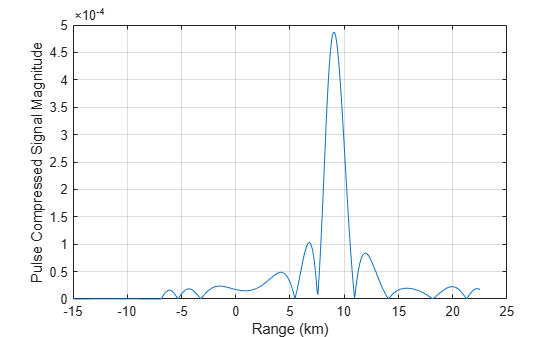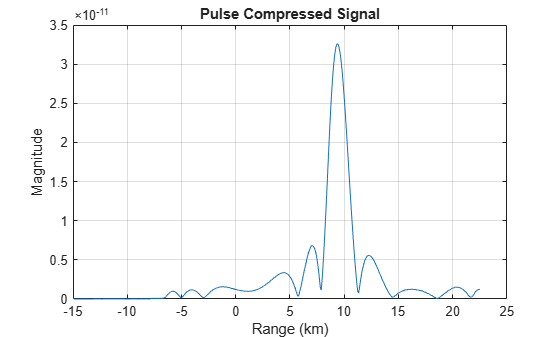# Frequency Agility in Radar, Communications, and EW Systems

This example shows how to model frequency agility in radar, communications, and electronic warfare systems to counter the effects of interference.

### Introduction

Active electronically-steered phased array systems can support multiple applications using the same array hardware. These applications may include radar, EW, and communications. However, the RF environments that these types of systems operate in are complex and sometimes hostile. For example, a repeater jammer can repeat the received radar signal and retransmit it to confuse the radar. In some literature, this is also called spoofing. Frequency agility can be an effective technique to counter the signals generated from interference sources and help support effective operations of these systems.

In this example, we first set up a scenario with a stationary monostatic radar and a moving aircraft target. The aircraft then generates a spoofing signal which confuses the radar. Once the radar detects the jamming source, frequency agility techniques can be employed which allows the radar to overcome the interference.

### System Simulation In an Interference Free Environment

Assume an X-band monostatic radar at the origin.

```fc = 10e9; % Define frequency of operation for X-band Radar fs = 2e6; % Define sample rate for the systems c = 3e8; lambda = c/fc; radar_pos = [0;0;0]; radar_vel = [0;0;0];```

The radar receiver, which can also function as an EW receiver, is a 64-element (8-by-8) Uniform Rectangular Array (URA) with half-wavelength spacing.

```% Array specification for the radar taper = taylorwin(8); taperURA = taper.*taper'; antenna = phased.URA('Element',phased.CosineAntennaElement, ... 'Size',[8 8],'ElementSpacing',[lambda/2 lambda/2], ... 'Taper',taperURA);```

The beam pattern of the array is shown in the following figure.

`pattern(antenna,fc,'Type','powerdb')````wav = phased.LinearFMWaveform('SampleRate',fs, ... 'PulseWidth', 10e-5, 'SweepBandwidth', 1e5,'PRF',4000, ... 'FrequencyOffsetSource','Input port'); tx = phased.Transmitter('Gain',20,'PeakPower',500); txArray = phased.WidebandRadiator('SampleRate',fs, ... 'Sensor',antenna,'CarrierFrequency', fc); rxArray = phased.WidebandCollector('SampleRate',fs, ... 'Sensor',antenna,'CarrierFrequency',fc); rxPreamp = phased.ReceiverPreamp('Gain',10,'NoiseFigure',5, ... 'SampleRate',fs);```

Specifythe environment and target. This example uses wideband propagation channels to propagate waveforms with different carrier frequencies.

```target = phased.RadarTarget('MeanRCS',100,'OperatingFrequency',fc); target_pos = [8000;1000;1000]; target_vel = [100;0;0]; % Outgoing and incoming channel envout = phased.WidebandFreeSpace('TwoWayPropagation',false, ... 'SampleRate', fs,'OperatingFrequency',fc,'PropagationSpeed',c); envin = phased.WidebandFreeSpace('TwoWayPropagation',false, ... 'SampleRate', fs,'OperatingFrequency',fc,'PropagationSpeed',c);```

This example uses two one-way propagation channels because the jamming signal only propagates through the return channel.

```rng(2017); [tgtRng, tgtAng] = rangeangle(target_pos, radar_pos); x = wav(0); % waveform xt = tx(x); % transmit xtarray = txArray(xt, tgtAng); % radiate yp = envout(xtarray,radar_pos,target_pos,radar_vel,target_vel); % propagate yr = target(yp); % reflect ye = envin(yr,target_pos,radar_pos,target_vel,radar_vel); % propagate yt = rxArray(ye,tgtAng); % collect yt = rxPreamp(yt); % receive```

Estimate the direction of arrival using a 2-D beam scan and use the estimated azimuth and elevation angles to direct the beamformer.

```estimator = phased.BeamscanEstimator2D('SensorArray',antenna,... 'DOAOutputPort',true, ... 'OperatingFrequency', fc, ... 'NumSignals',1, ... 'AzimuthScanAngles',-40:40, ... 'ElevationScanAngles',-60:60); [~,doa] = estimator(yt); beamformer = phased.SubbandPhaseShiftBeamformer('SensorArray',antenna, ... 'OperatingFrequency',fc,'DirectionSource','Input port', ... 'SampleRate',fs,'WeightsOutputPort',true); [ybf,~] = beamformer(yt,doa);```

Pass the beamformed signal through a matched filter and detector.

```mfcoeff1 = getMatchedFilter(wav); mf1 = phased.MatchedFilter('Coefficients',mfcoeff1);```

The following figure shows that the target produces a dominant peak in the received signal.

```y1 = mf1(ybf); nSamples = wav.SampleRate/wav.PRF; t = ((0:nSamples-1)-(numel(mfcoeff1)-1))/fs; r = t*c/2; plot(r/1000,abs(y1),'-') grid on xlabel('Range (km)') ylabel('Pulse Compressed Signal Magnitude')```### Signal Analysis of Target and Jammer

The radar works very well in the previous section. However, in a complex environment, interference can affect the radar performance. The interference may be from other systems, such as wireless communication signals, or jamming signals. Modern radar systems must be able to operate in such environments.

A phased array radar can filter out interference using spatial processing. If the target and the jamming source are well-separated in angular space, beamforming can be an effective way to suppress the jammer. More details can be found in the Array Pattern Synthesis Part I: Nulling, Windowing, and Thinning example.

This example focuses on the situation where the target and the interference are closely located so that spatial processing cannot be used to separate the two. Consider the case where the target aircraft can determine the characteristics of the signal transmitted from the radar and use that information to generate a pulse that will confuse the radar receiver. This is a common technique used in jamming or spoofing to draw the radar away from the true target.

Display the detected signal characteristics.

```pw = (pulsewidth(abs(yp), fs)); prf = round(1/pulseperiod(abs([yp;yp]), fs)); bw = obw(yp,fs,[],95); fprintf('Waveform Characteristics:\n');```
```Waveform Characteristics: ```
`fprintf('Pulse width:\t\t%f\n',pw);`
```Pulse width: 0.000100 ```
`fprintf('PRF:\t\t\t%f\n',prf);`
```PRF: 4000.000000 ```
`fprintf('Sweep bandwidth:\t%f\n',bw);`
```Sweep bandwidth: 112041.098255 ```

The jammer needs time to do this analysis and prepare the jamming signal, so it is hard to create an effective spoofing signal right away. But generally within several pulse intervals the jamming signal is ready and the jammer can put it in an arbitrary position in a pulse to make the spoofing target look closer or farther compared to the true target. It is also worth noting that with the latest hardware, the time needed to estimate the characteristics of the signal decreases dramatically.

Assume the jammer wants to put the signal at about 5.5 km, the jammer could transmit the jamming signal at the right moment to introduce the corresponding delay. In addition, because this is a one way propagation from the jammer to the radar, the required power is much smaller. This is indeed what makes jamming very effective as it does not require much power to blind the radar.

```jwav = phased.LinearFMWaveform('SampleRate',fs, ... 'PulseWidth',pw,'SweepBandwidth',bw,'PRF',prf); xj = jwav(); Npad = ceil(5500/(c/fs)); xj = circshift(xj,Npad); % pad zero to introduce corresponding delay txjam = phased.Transmitter('Gain',0,'PeakPower',5); xj = txjam(xj); ye = envin(yr+xj,target_pos,radar_pos,target_vel,radar_vel); yt = rxArray(ye,tgtAng); yt = rxPreamp(yt); ybfj = beamformer(yt,doa); y1j = mf1(ybfj); % Jammer plus target return plot(r/1000,abs(y1j)) grid on xlabel('Range (km)') ylabel('Magnitude') title('Pulse Compressed Return From Target And Jammer')```The received signal now contains both the desired target return and the jamming signal. In addition, the jamming signal appears to be closer. Therefore, the radar is more likely to lock in on the closest target thinking that it is the most prominent threat and spend less resources on the true target.

### Frequency Agility to Counter Interference

In the following situation, assume that the transmitted signal hops 500 kHz from the original carrier frequency of 10 GHz. Therefore, the new waveform signal can be represented as follows.

```deltaf = fs/4; xh = wav(deltaf); % hopped signal ```

Ths figure shows the spectrogram of both the original signal and the hopped signal. Note that the hopped signal is a shift in the frequency domain compared to the original signal.

`pspectrum(x+xh,fs,'spectrogram')`Using a similar approach outlined in the earlier sections, the radar echo can be simulated using the new waveform. Note that since jammer is not aware of this hop, the jamming signal is still the same.

```xth = tx(xh); xtharray = txArray(xth, tgtAng); yph = envout(xtharray,radar_pos,target_pos,radar_vel,target_vel); yrh = target(yph); yeh = envin(yrh+xj,target_pos,radar_pos,target_vel,radar_vel); yth = rxArray(yeh,tgtAng); yth = rxPreamp(yth); ybfh = beamformer(yth,doa);```

Because the hopping schedule is known to the radar, the signal processing algorithm could use that information to extract only the frequency band that around the current carrier frequency. This helps reject the interference at other bands and also improves the SNR since the noise from other bands is suppressed. In addition, when the waveform hops, the matched filter needs to be updated accordingly.

Now apply the corresponding bandpass filters and matched filters to the received signal.

First, create a bandpass filter using the signal bandwidth of the signal.

`buttercoef = butter(9,bw/fs);`

Then modulate the resulting bandpass filter with a carrier to obtain the bandpass filter around that carrier frequency.

`bf2 = buttercoef.*exp(1i*2*pi*deltaf*(0:numel(buttercoef)-1)/fs);`

Similarly, the modulate the matched filter coefficient.

```mfcoeff2 = getMatchedFilter(wav,'FrequencyOffset',deltaf); mf2 = phased.MatchedFilter('Coefficients',mfcoeff2); % extract bands and apply matched filters yb2 = mf2(filter(bf2(:),1,ybfh)); % plot the matched filtered signal plot(r/1000,abs(yb2)) grid on xlabel('Range (km)') ylabel('Magnitude') title('Pulse Compressed Signal')```This figure shows that with the adoption of frequency hopping, the target echo and the jamming signal can be separated. Since the jammer is still in the original band, only the true target echo appears in the new frequency band that the waveform currently occupies, thus suppressing the impact of the jammer.

### Summary

This example shows that adopting frequency agility can help counter the jamming effect in a complex RF environment. The example simulates a system with a frequency hopping waveform and verifies that this technique helps the radar system to identify the true target echo without being confused by the jamming signal.